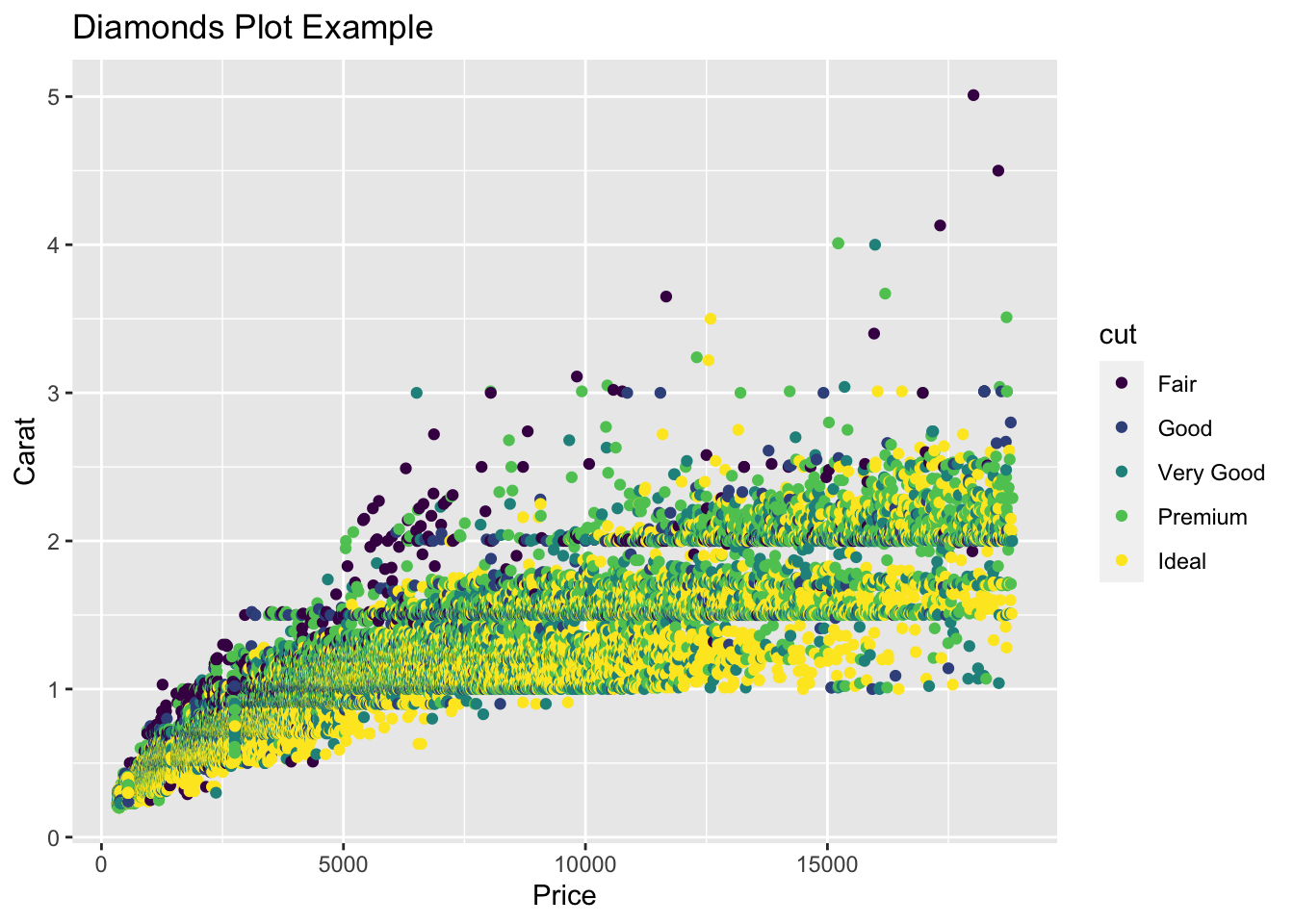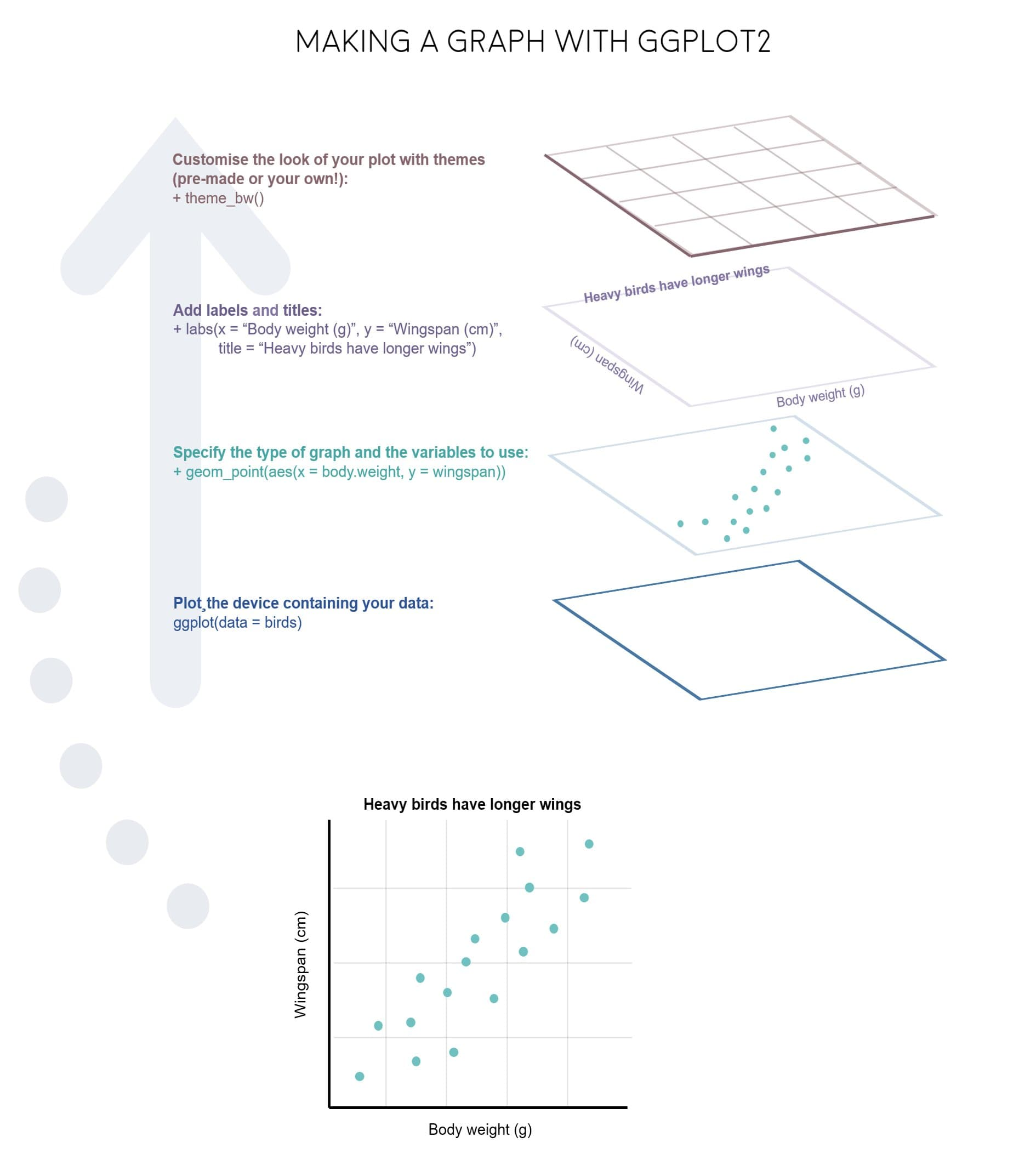# Learning Material

As mentioned within the session setup, load the following packages using the `library()` function.

``````  library(tidyverse)
library(RColorBrewer)
library(ghibli)
library(palettetown)``````

Introduction

Example Plot:

To give you an example of how to generally plot in R, using `ggplot2`, we can examine the diamonds data set. This is an extremely common example, but is useful to understand how to structure of how to plot.

``````# Chunk 1
ggplot(data = diamonds,
mapping = aes(x = price,
y = carat,
colour = cut)) +
# Chunk 2
geom_point() +
# Chunk 3
labs(title = "Diamonds Plot Example",
x = "Price",
y = "Carat")``````We can break down the code for this plot into three Chunks.

• Chunk 1: `ggplot()` function, this is the core part of any visualization function, and typically contains information such as specification of the data to be used, and the mapping aesthetics. These details however can also be included within Chunk 2.
• Chunk 2: `geom` specification, details which type of plot you would like to plot. In this case, we are plotting a point chart, or scatter plot.
• Chunk 3: Additional Details, such as `labs()`. In this case, specifying the labels which should be included alongside your plot.

These three components (that is the ggplot function, the geom specification and additional details) are core components of any data visualization. And can be summed up also in the diagram below.Alternatively, this code can be written as so, and produce the same results.

``````# Chunk 1
ggplot() +
# Chunk 2
geom_point(data = diamonds,
mapping = aes(x = price,
y = carat,
colour = cut)) +
# Chunk 3
labs(title = "Diamonds Plot Example",
x = "Price",
y = "Carat")``````

Understanding that there are multiple methods of achieving the same result is incredibly important, especially during programming in R.

Layered Coding

As you can see, these visualizations are made up of lots of smaller components. These changeable aspects include:

geom functions

• `geom_line()` - line charts
• `geom_point()` - scatter plots
• `geom_polygon()` - shape diagrams

Aesthetic Descriptors These are used within the function, `aes = mapping()`

• Fill = - inside/fill colour
• Colour = - Border colour
• Alpha = - Transparency Level (0 -> 1)
• Linetype = - Line Type / Border Type
• Size = - Border thickness / Point Size
• Shape = - Point Shape

Themes

• `theme()` - base theme
• `theme_bw()` - black and white theme
• `theme_void()` - empty theme (no scales, legends etc)
• `theme_minimal()` - minimal theme

Scales

• `labs()`, `xlab()`, `ylab()`, `ggtitle()` - Labels
• `lims()`, `xlim()`, `ylim()` - Scale Limits
• `scale_x_log10()`, `scale_y_log10()` - Log Scales

Coordinate Systems

• `coord_cartesian()` - Cartesian Coordinate System
• `coord_polar()` - Polar Coordinate System

Understanding Polar Coordinates

• Coordinates on the x-axis indicate the circular movement.

• Coordinates on the y-axis indicate the distance of the points from the origin.

• The origin of the plot is defined as the centre of the plot

• The plot begins and ends at the top of the plot (before rotating right)

Incorporating colour

When it comes to applying colour to the plots you produce, there again are multiple ways in which these can be defined. This includes:

Base R:

• Blue: `"blue"`
• Red: `"red"`

Hex/RGB Code:

• Blue: #0074D9
• Red: #FF4136

Palettes from R Packages

• RColorBrewer
• ghibli (colours inspired by Studio Ghibli Films)
• palettetown (colour inspired by pokemon)

Practical Exercises